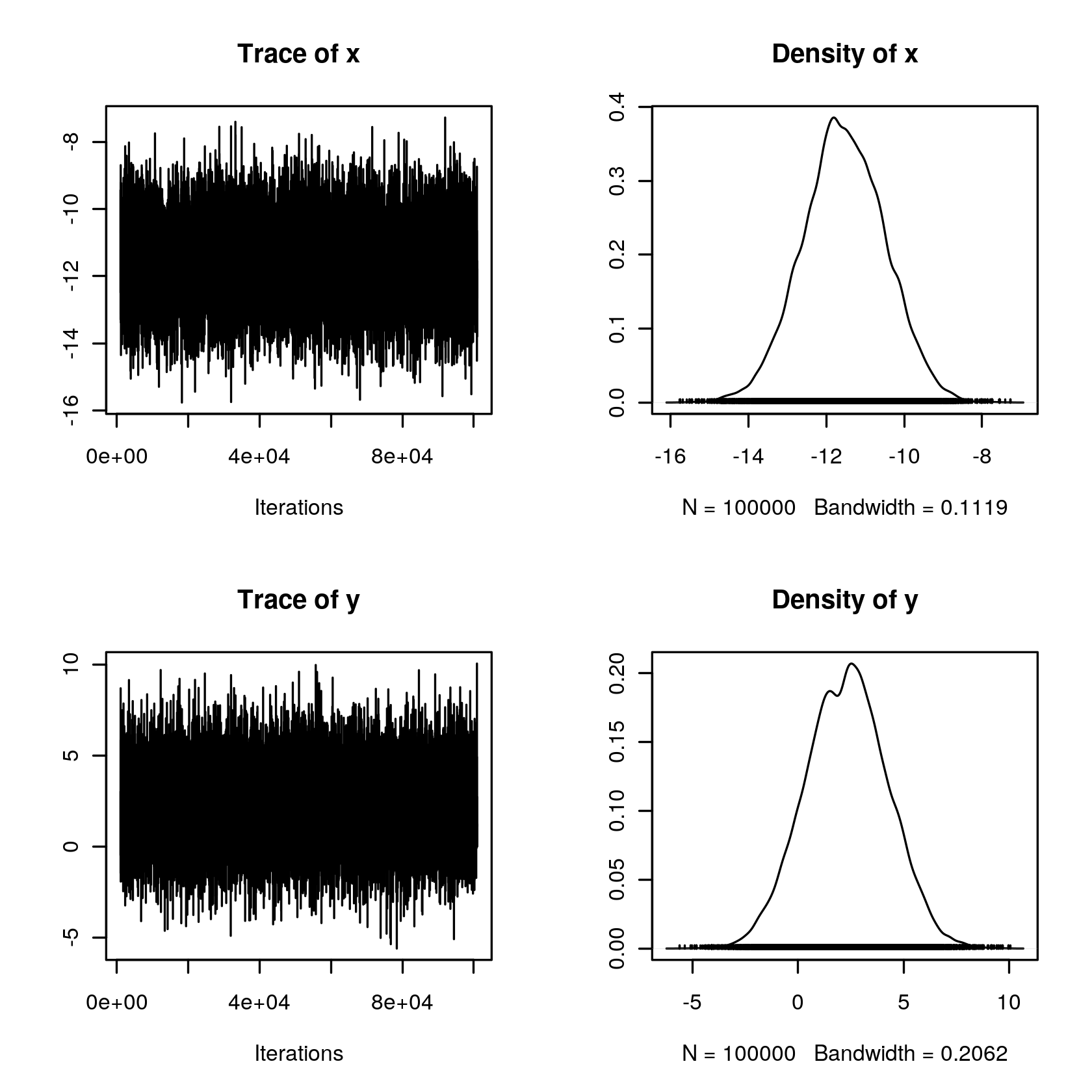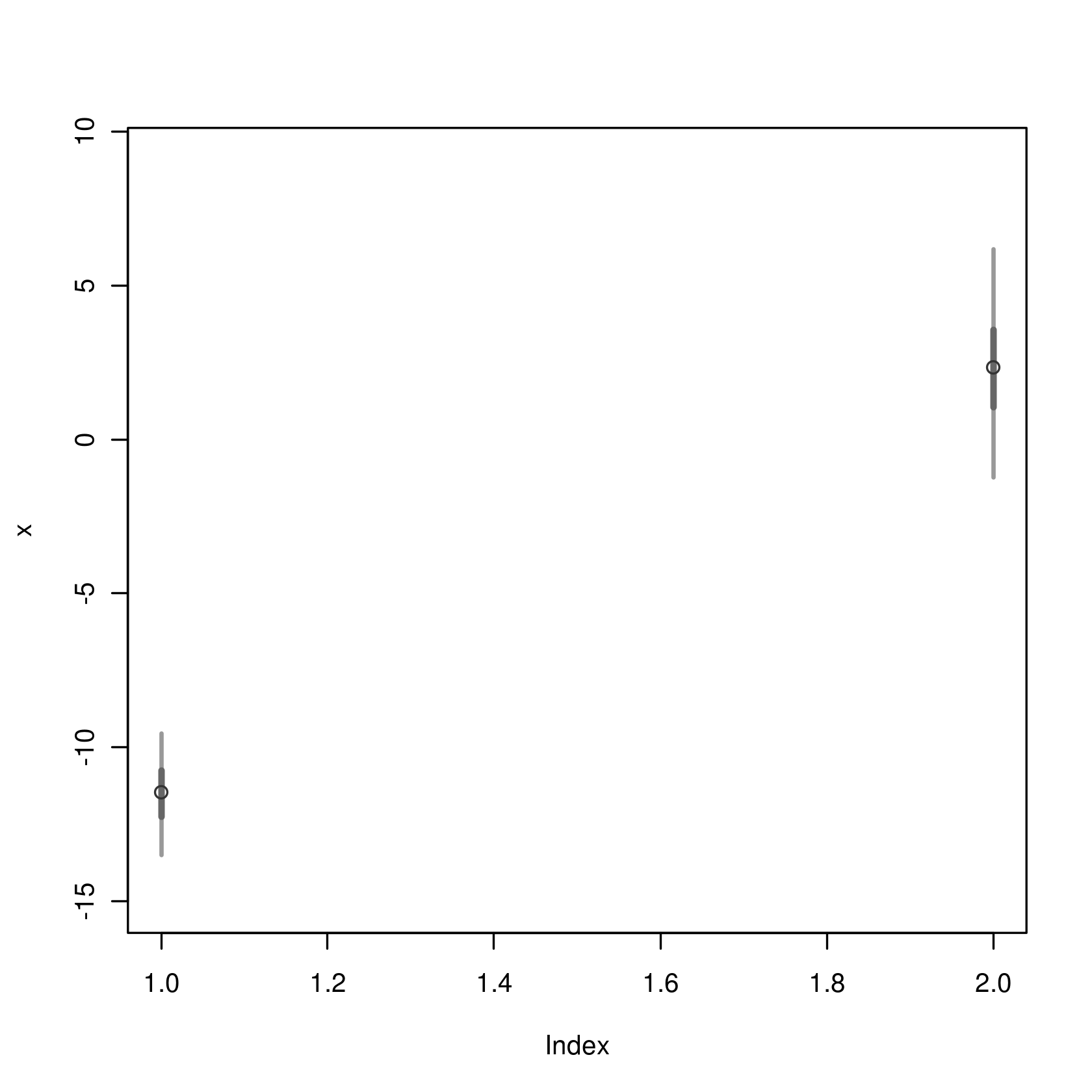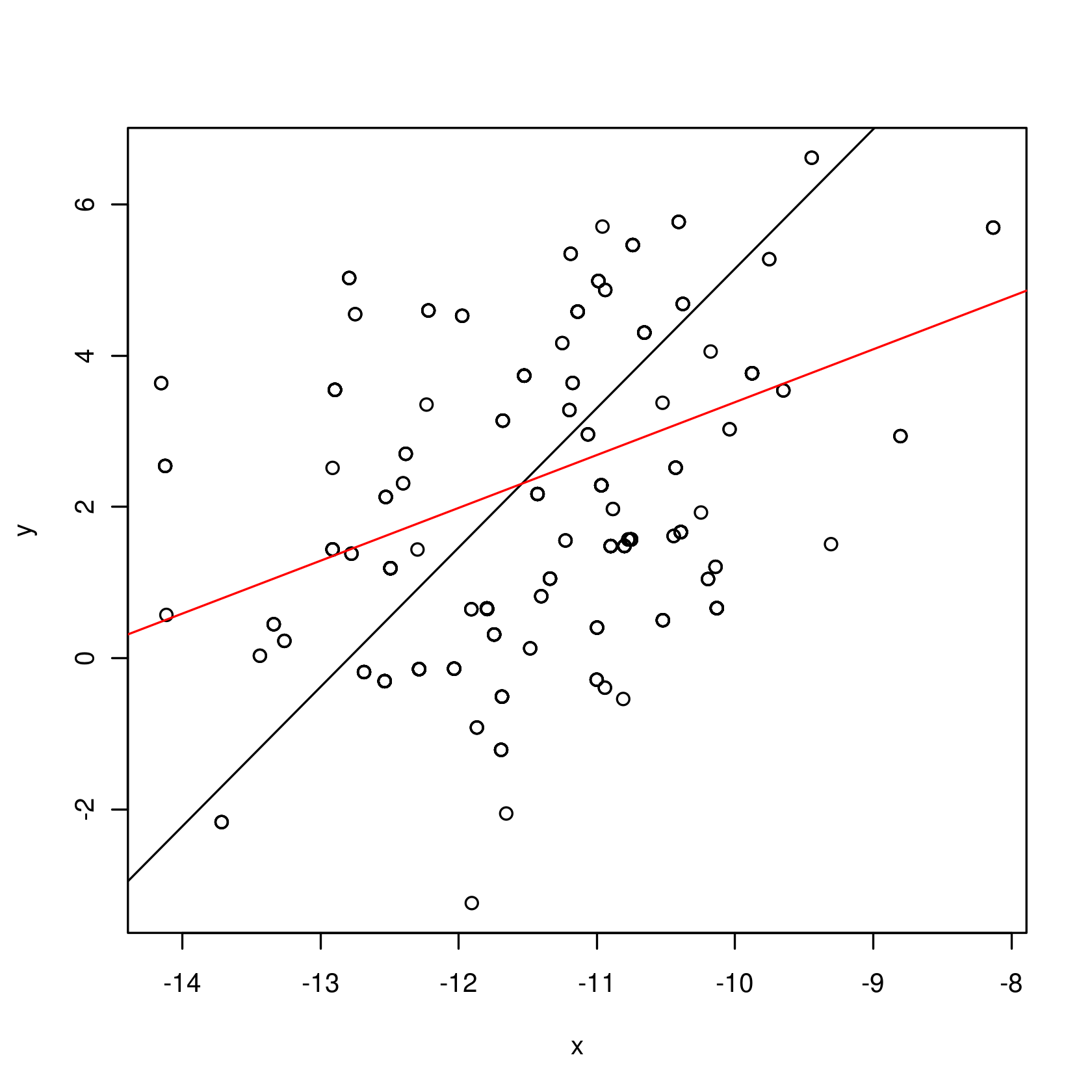# Sampling a bivariate Gaussian by Metropolis

 Author Carlos Rodriguez Date 2017-11-15T16:33:45 Project e17ee2b4-4b6f-4284-835f-dc56e2715236 Location assignments/bigauss.sagews Original file bigauss.sagews# Sampling a bivariate Gaussian by MetropolisHere is the problem: Get samples from a pdf   $p(x,y)$ such that,

$$\log p(x,y) = -a x^{2} - b y^{2} + c x y + d x + e y + C.$$ This is the pdf of a non singular bivariate Gaussian provided that:

1) Is proper over the euclidean plane $R^{2}$ for which you need $a>0, b>0$.

2) It does not concentrate its mass on a line; For which you need $4 ab > c^{2}$ (see below).

Under these condtions, the plain Metropolis MCMC below will converge and one can estimate the 5 parameters: $$\theta = (\mu_{x},\mu_{y},\sigma_{x},\sigma_{y},\rho)$$ from the simulated samples. Thus, in fact, providing an approximate solution to a system of 5 non linear equations in 5 unknowns!. We use Sage to find the exact solution for this system and hence check that the MCMC gives precise approximations. Feel free to play around with the abc's and the parameters of the Metropolis MCMC by changing their values in the next cell.

%r# sampling from log p(x,y) = -ax^2-by^2+cxy+dx+ey + C.################################################################ Pick values. For solution to exist Need: a>0, b>0, 4ab > c^2.a <- 2b <- 0.15c <- 0.8d <- -12e <- 3################################################################################################################################# MCMC parameters:n.samples <- 1e5x0y0 <- c(0,0)sigma.proposal.x <- 2sigma.proposal.y <- 5###################################################################  no need to change anything below this point.###################################################################

Note: If you change anything in the previous cell, make sure you hit [evaluate] there AND the next cell.

### This is where the samples are obtained.

If R complains about MCMCpack it means that you need to install it in your system. (Just go to an empty cell and type: "r.install_packages('MCMCpack')" and, provided you have the perms and you do have the perms when you are running your own Sage as an admin, you are done.) The functions in the MCMCpack are compiled C so they are usually very fast. Try getting a healthy acceptance rate of about 17%  (Yes that low is best....) by changing the SDs of the proposals for x and y (sigma.proposal.x and sigma.proposal.y above).
%rrequire(MCMCpack)# test values:# Need: a>0, b>0, 4ab > c^2# if there is a solution it is given by:# sol()a <- 0.5; b <- 0.15; c <- 0.2; d <- -12; e <- 3abc <- c(a,b,c,d,e)logf <- function(theta,alpha) {    x <- theta    y <- theta    -a*x^2-b*y^2+c*x*y+d*x+e*y}xy <- MCMCmetrop1R(logf,theta.init= x0y0, mcmc= n.samples,tune=3,burnin = 1000,alpha=abc)colnames(xy) <- c("x","y")summary(xy)r <- cor(xy[,1],xy[,2])print(paste("cor(x,y)=",r))
## ## Markov Chain Monte Carlo Package (MCMCpack)
## Copyright (C) 2003-2017 Andrew D. Martin, Kevin M. Quinn, and Jong Hee Park
## ## Support provided by the U.S. National Science Foundation
## (Grants SES-0350646 and SES-0350613) ##
@@@@@@@@@@@@@@@@@@@@@@@@@@@@@@@@@@@@@@@@@@@@@@@@@@@@@@@@@ The Metropolis acceptance rate was 0.16741 @@@@@@@@@@@@@@@@@@@@@@@@@@@@@@@@@@@@@@@@@@@@@@@@@@@@@@@@@ Iterations = 1001:101000 Thinning interval = 1 Number of chains = 1 Sample size per chain = 1e+05 1. Empirical mean and standard deviation for each variable, plus standard error of the mean: Mean SD Naive SE Time-series SE x -11.543 1.056 0.003339 0.01080 y 2.307 1.945 0.006151 0.01986 2. Quantiles for each variable: 2.5% 25% 50% 75% 97.5% x -13.611 -12.2452 -11.547 -10.821 -9.489 y -1.499 0.9768 2.332 3.612 6.068  "cor(x,y)= 0.379114557940978"## Finding the exact solution with Sage

We are looking for a bivariate Gaussian with parameter $\theta$ identical to the $p(x,y)$ with given values $(a,b,c,d,e)$. The $\theta$'s and the $abc$'s are just two different systems of coordinates for the bivariate Gaussians. Recall the equation for the pdf of a bivariate Gaussian $N$ with mean vector $\mu$ and variance-covariance matrix $\Sigma$ is such that, $$\log N(x,y) = -\frac{1}{2} (x-\mu_{x},y-\mu_{y})\Sigma^{-1} \left(\begin{array}{r} x-\mu_{x} \\ y-\mu_{y} \end{array}\right) + K$$ where, $$\Sigma = \left(\begin{array}{rr} \sigma_{x}^{2} & \rho \sigma_{x} \sigma_{y} \\ \rho \sigma_{x} \sigma_{y} & \sigma_{y}^{2} \end{array}\right)$$ The 5 equations  for the components of $\theta$ are obtained by imposing that for all $(x,y)$ on the plane, $$-K + \log N(x,y) = -C + \log p(x,y)$$ Therefore, we need to equate the coefficients of the two quadratic forms. In the following cell we let Sage do the algebra for us.
%typeset_mode True%var a,b,c,d,e,x,y,mu_x,mu_y,sigma_x,sigma_y,rhoload('https://omega0.xyz/omega8008/Sim.py')f= -a*x^2-b*y^2+c*x*y+d*x+e*ya = -f.coefficient(x^2)b = -f.coefficient(y^2)c = f.coefficient(x).coefficient(y)d = (f-c*x*y).coefficient(x)e = (f-c*x*y).coefficient(y)Sigma = matrix(2,2,[sigma_x^2,rho*sigma_x*sigma_y,rho*sigma_x*sigma_y,sigma_y^2])xy = vector([x,y])mu = vector([mu_x,mu_y])z=(-(1/2)*(xy-mu)*(~Sigma)*((xy-mu)).column())z = expand(z)exy = Sim(z.coefficient(x).coefficient(y))eqx= Sim((z-exy*x*y).coefficient(x))eqy= Sim((z-exy*x*y).coefficient(y))exx = Sim(z.coefficient(x^2))eyy = Sim(z.coefficient(y^2))eqs = [exx== -a, eyy== -b, exy== c, eqx==d,eqy==e]#sol = solve(eqs,mu_x,mu_y,sigma_x,sigma_y,rho)#sabc = [[eq.subs(abc) for eq in sol[i]] for i in range(len(sol))]#r = [sabc[i] for i in range(len(sabc)) if (sabc[i].rhs()>0)and(sabc[i].rhs()>0)]#rassume(a>0,b>0,4*a*b-c^2>0)sol1 = solve([exy-c,exx+a,eyy+b],rho,sigma_x,sigma_y)# simplifyssol = [{h.lhs():Sim(h.rhs()) for h in s0} for s0 in sol1]Sol = ssolsmu = solve([eqx-d,eqy-e],mu_x,mu_y)sss = [{h.lhs():Sim(h.rhs()) for h in s0} for s0 in smu]Sol.update(sss)Sol[mu_x] = Sim(Sol[mu_x].subs(Sol))Sol[mu_y] = Sim(Sol[mu_y].subs(Sol))eqsSol
Using _k = _k_default = 5, TL = TL_all[0:-1] = ['_rr', '_dc', '_ps', '_el', '_sl', '_fs', '_Snj', '_Sdj', '_cb', '_xp', '_sr', '_er', '_es', '_fa', '_pf', '_mrs', '_pmrs']
[$\displaystyle \frac{1}{2 \, {\left(\rho^{2} - 1\right)} \sigma_{x}^{2}} = -a$, $\displaystyle \frac{1}{2 \, {\left(\rho^{2} - 1\right)} \sigma_{y}^{2}} = -b$, $\displaystyle -\frac{\rho}{{\left(\rho^{2} - 1\right)} \sigma_{x} \sigma_{y}} = c$, $\displaystyle \frac{\mu_{y} \rho \sigma_{x} - \mu_{x} \sigma_{y}}{{\left(\rho^{2} - 1\right)} \sigma_{x}^{2} \sigma_{y}} = d$, $\displaystyle \frac{\mu_{x} \rho \sigma_{y} - \mu_{y} \sigma_{x}}{{\left(\rho^{2} - 1\right)} \sigma_{x} \sigma_{y}^{2}} = e$]
$\displaystyle \left\{\rho : -\frac{c}{2 \, \sqrt{a} \sqrt{b}}, \sigma_{y} : \sqrt{2} \sqrt{\frac{a}{4 \, a b - c^{2}}}, \sigma_{x} : \sqrt{2} \sqrt{\frac{b}{4 \, a b - c^{2}}}, \mu_{y} : \frac{c d + 2 \, a e}{4 \, a b - c^{2}}, \mu_{x} : \frac{2 \, b d - c e}{4 \, a b - c^{2}}\right\}$

# equations:eqs
[$\displaystyle \frac{1}{2 \, {\left(\rho^{2} - 1\right)} \sigma_{x}^{2}} = -a$, $\displaystyle \frac{1}{2 \, {\left(\rho^{2} - 1\right)} \sigma_{y}^{2}} = -b$, $\displaystyle -\frac{\rho}{{\left(\rho^{2} - 1\right)} \sigma_{x} \sigma_{y}} = c$, $\displaystyle \frac{\mu_{y} \rho \sigma_{x} - \mu_{x} \sigma_{y}}{{\left(\rho^{2} - 1\right)} \sigma_{x}^{2} \sigma_{y}} = d$, $\displaystyle \frac{\mu_{x} \rho \sigma_{y} - \mu_{y} \sigma_{x}}{{\left(\rho^{2} - 1\right)} \sigma_{x} \sigma_{y}^{2}} = e$]
# solution:Sol
$\displaystyle \left\{\rho : -\frac{c}{2 \, \sqrt{a} \sqrt{b}}, \sigma_{y} : \sqrt{2} \sqrt{\frac{a}{4 \, a b - c^{2}}}, \sigma_{x} : \sqrt{2} \sqrt{\frac{b}{4 \, a b - c^{2}}}, \mu_{y} : \frac{c d + 2 \, a e}{4 \, a b - c^{2}}, \mu_{x} : \frac{2 \, b d - c e}{4 \, a b - c^{2}}\right\}$

### Sage, you are the Man!

Thank you Sage. I am glad I did not have to do it. In the next cell we push the simplicfication a bit further. Notice that it should now be clear why we need $$a > 0, b > 0, 4ab > c^2$$ so that we are not dividing by zero in the formulas for the change of variables below.

### Back to R

Now that we have the exact solution we can write a little "sol()" function in R with the formulas provided by Sage. We can now check how close the Monte Carlo solution actually was.
%r# test values:#a <- 0.5; b <- 0.15; c <- 0.2; d <- -12; e <- 3#abc <- c(a,b,c,d,e)# Need: a>0, b>0, 4ab > c^2# if there is a solution it is given by:# sol()# The true solution:sol <- function(alpha=abc) {    a <- alpha    b <- alpha    c <- alpha    d <- alpha    e <- alpha    return (list(mu_x = (2*b*d + c*e)/(4*a*b - c^2),               mu_y = (2*a*e + c*d)/(4*a*b - c^2),               sigma_x = sqrt(2*b/(4*a*b - c^2)),               sigma_y = sqrt(2*a/(4*a*b - c^2)),               rho = 1/2*c*sqrt(1/(a*b))))}sol(abc)
$mu_x -11.5384615384615$mu_y
2.30769230769231
$sigma_x 1.07417231105915$sigma_y
1.96116135138184
$rho 0.365148371670111 Let's see that the MCMC was healthy, Using rv. %rrequire(rv)XY <- rvsims(xy,n.sims=nrow(xy))print(XY)plot(XY) Loading required package: rv Loading required package: parallel name mean sd 1% 2.5% 25% 50% 75% 97.5% 99% sims  x -11.5 1.1 -14.0 -13.6 -12.25 -11.5 -10.8 -9.5 -9.1 100000  y 2.3 1.9 -2.1 -1.5 0.98 2.3 3.6 6.1 6.8 100000Do the samples look like a bivariate Gaussian with the$\theta\$ parameters?

%rk <- 1000plot(xy[k:(k+500),])X <- xy[,1]; Y <- xy[,2]mx <- mean(X); my <- mean(Y); sx <- sd(X); sy <- sd(Y); r <- cor(X,Y)# sd line:s <- sign(r)*sy/sxabline(b=s,a=my-s*mx)# regression line:abline(b=r*sy/sx,a=my-mx*r*sy/sx,col="red")# Summarizing

mu_x mu_y sigma_x sigma_y rho true -11.538 2.31 1.074 1.96 0.3650 mcmc -11.543 2.31 1.056 1.95 0.3790 ± 0.011 0.02 0.011 0.02 0.0032